Functions versus Relations (page 1 of 2)

Sections: Functions versus relations, Domain and rangeThere are different ways of looking at functions. We will consider a few.  But first, we need to discuss some terminology.

A "relation" is just a relationship between sets of information. Think of all the people in one of your classes, and think of their heights. The pairing of names and heights is a relation. In relations and functions, the pairs of names and heights are "ordered", which means one comes first and the other comes second. To put it another way, we could set up this pairing so that either you give me a name, and then I give you that person's height, or else you give me a height, and I give you the names of all the people who are that tall. The set of all the starting points is called "the domain" and the set of all the ending points is called "the range." The domain is what you start with; the range is what you end up with. The domain is the x's; the range is the y's. (I'll explain more on the subject of determining domains and ranges later.)

A function is a "well-behaved" relation. Just as with members of your own family, some members of the family of pairing relationships are better behaved than other. (Warning: This means that, while all functions are relations, since they pair information, not all relations are functions. Functions are a sub-classification of relations.) When we say that a function is "a well-behaved relation", we mean that, given a starting point, we know exactly where to go; given an x, we get only and exactly one y.

Let's return to our relation of your classmates and their heights, and let's suppose that the domain is the set of everybody's heights. Let's suppose that there's a pizza-delivery guy waiting in the hallway. And all the delivery guy knows is that the pizza is for the student in your classroom who is five-foot-five. Now let the guy in. Who does he go to? What if nobody is five-foot-five? What if there are six people in the room that are five-five? Do they all have to pay? What if you are five-foot-five? And what if you're out of cash? And allergic to anchovies? Are you still on the hook? Ack! What a mess!

The relation "height indicates name" is not well-behaved. It is not a function. Given the relationship (x, y) = (five-foot-five person, name), there might be six different possibilities for y = "name". For a relation to be a function, there must be only and exactly one y that corresponds to a given x. Here are some pictures of this: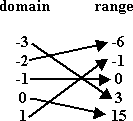This is a function. You can tell by tracing from each x to each y. There is only one y for each x; there is only one arrow coming from each x.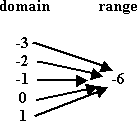Ha! Bet I fooled some of you on this one! This is a function! There is only one arrow coming from each x; there is only one y for each x. It just so happens that it's always the same y for each x, but it is only that one y. So this is a function; it's just an extremely boring function!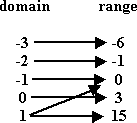This one is not a function: there are two arrows coming from the number 1; the number 1 is associated with two different range elements. So this is a relation, but it is not a function.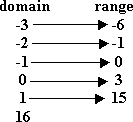Okay, this one's a trick question. Each element of the domain that has a pair in the range is nicely well-behaved. But what about that 16? It is in the domain, but it has no range element that corresponds to it! This won't work! So then this is not a function. Heck, it ain't even a relation!

Now YOU try!

The "Vertical Line Test"

Looking at this function stuff graphically, what if we had the relation that consists of a set containing just two points: {(2, 3), (2, –2)}? We already know that this is not a function, since x = 2 goes to each of y = 3 and y = –2.   Copyright © Elizabeth Stapel 1999-2011 All Rights Reserved

 If we graph this relation, it looks like:Notice that you can draw a vertical line through the two points, like this: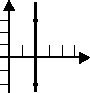This characteristic of non-functions was noticed by I-don't-know-who, and was codified in "The Vertical Line Test": Given the graph of a relation, if you can draw a vertical line that crosses the graph in more than one place, then the relation is not a function. Here are a couple examples: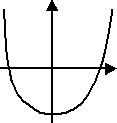This graph shows a function, because there is no vertical line that will cross this graph twice.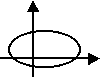This graph does not show a function, because any number of vertical lines will intersect this oval twice. For instance, the y-axis intersects (crosses) the line twice.

Now YOU try!

"Is it a function?" - Quick answer without the graph

Think of all the graphing that you've done so far. The simplest method is to solve for "y =", make a T-chart, pick some values for x, solve for the corresponding values of y, plot your points, and connect the dots, yadda, yadda, yadda. Not only is this useful for graphing, but this methodology gives yet another way of identifying functions: If you can solve for "y =", then it's a function. In other words, if you can enter it into your graphing calculator, then it's a function. The calculator can only handle functions. For example, 2y + 3x = 6 is a function, because you can solve for y:

2y + 3x = 6
2y =
3x + 6
y = (
3/2)x + 3

On the other hand, y2 + 3x = 6 is not a function, because you can not solve for a unique y: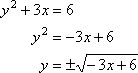I mean, yes, this is solved for "y =", but it's not unique. Do you take the positive square root, or the negative? Besides, where's the "±" key on your graphing calculator? So, in this case, the relation is not a function. (You can also check this by using our first definition from above. Think of "x =1". Then we get y23 = 6, so y2 = 9, and then y can be either –3 or +3. That is, if we did an arrow chart, there would be two arrows coming from x =1.)